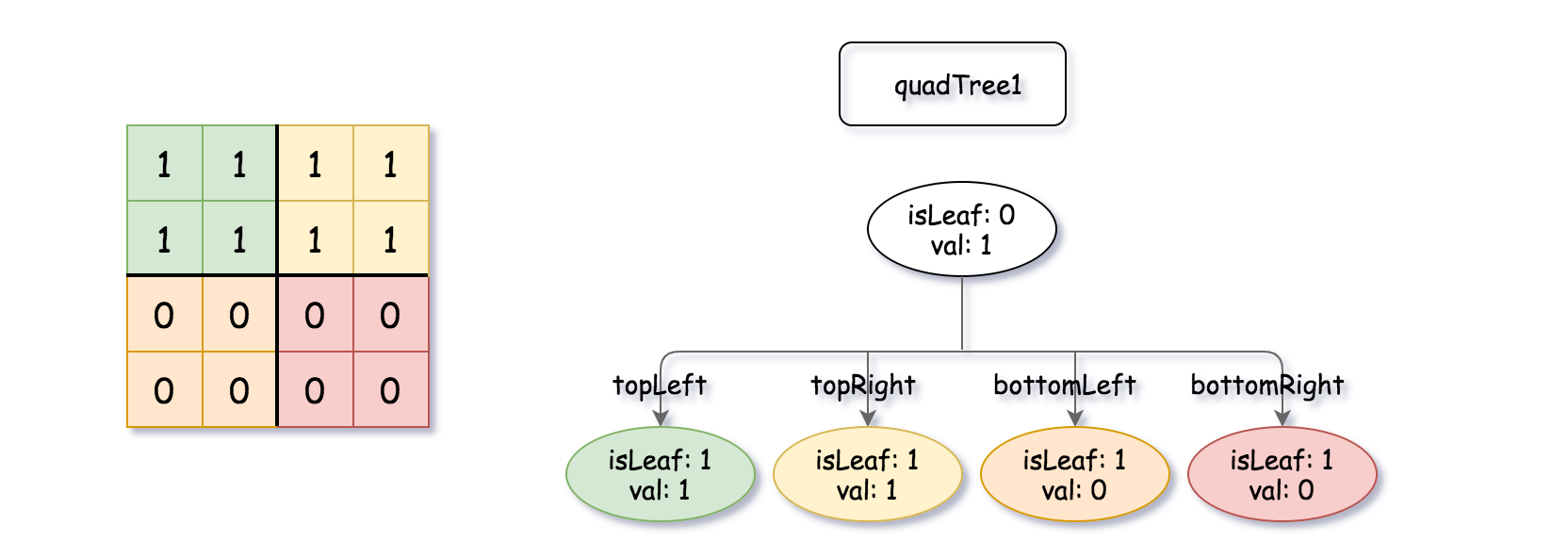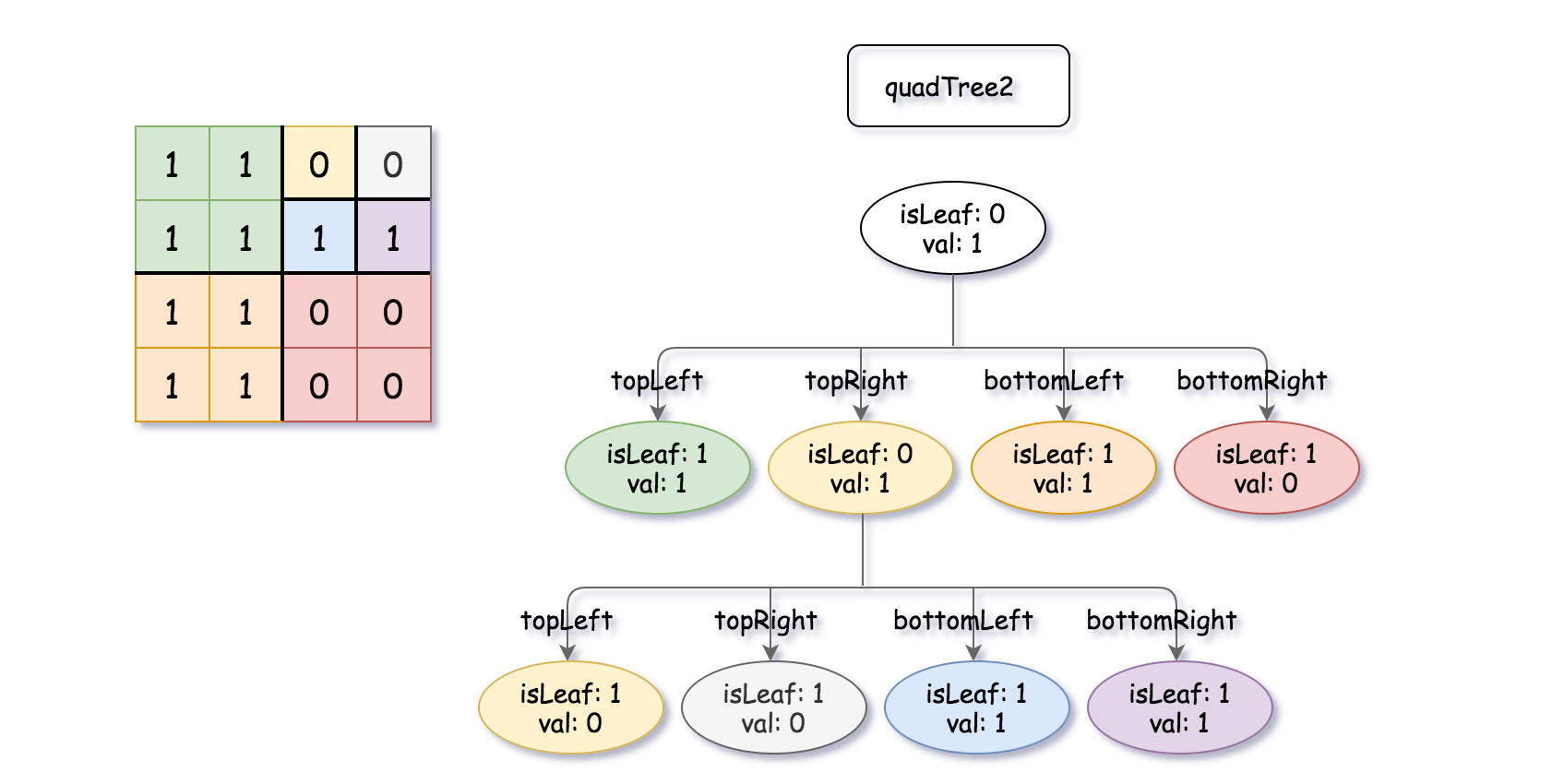## Algorithm

Problem Name: 558. Logical OR of Two Binary Grids Represented as Quad-Trees

A Binary Matrix is a matrix in which all the elements are either 0 or 1.

Given `quadTree1` and `quadTree2`. `quadTree1` represents a `n * n` binary matrix and `quadTree2` represents another `n * n` binary matrix.

Return a Quad-Tree representing the `n * n` binary matrix which is the result of logical bitwise OR of the two binary matrixes represented by `quadTree1` and `quadTree2`.

Notice that you can assign the value of a node to True or False when `isLeaf` is False, and both are accepted in the answer.

A Quad-Tree is a tree data structure in which each internal node has exactly four children. Besides, each node has two attributes:

• `val`: True if the node represents a grid of 1's or False if the node represents a grid of 0's.
• `isLeaf`: True if the node is leaf node on the tree or False if the node has the four children.
```class Node {
public boolean val;
public boolean isLeaf;
public Node topLeft;
public Node topRight;
public Node bottomLeft;
public Node bottomRight;
}```

We can construct a Quad-Tree from a two-dimensional area using the following steps:

1. If the current grid has the same value (i.e all `1's` or all `0's`) set `isLeaf` True and set `val` to the value of the grid and set the four children to Null and stop.
2. If the current grid has different values, set `isLeaf` to False and set `val` to any value and divide the current grid into four sub-grids as shown in the photo.
3. Recurse for each of the children with the proper sub-grid.If you want to know more about the Quad-Tree, you can refer to the wiki.

The input/output represents the serialized format of a Quad-Tree using level order traversal, where `null` signifies a path terminator where no node exists below.

It is very similar to the serialization of the binary tree. The only difference is that the node is represented as a list `[isLeaf, val]`.

If the value of `isLeaf` or `val` is True we represent it as 1 in the list `[isLeaf, val]` and if the value of `isLeaf` or `val` is False we represent it as 0.

Example 1:```Input: quadTree1 = [[0,1],[1,1],[1,1],[1,0],[1,0]]
Output: [[0,0],[1,1],[1,1],[1,1],[1,0]]
Explanation: quadTree1 and quadTree2 are shown above. You can see the binary matrix which is represented by each Quad-Tree.
If we apply logical bitwise OR on the two binary matrices we get the binary matrix below which is represented by the result Quad-Tree.
Notice that the binary matrices shown are only for illustration, you don't have to construct the binary matrix to get the result tree.```

Example 2:

```Input: quadTree1 = [[1,0]], quadTree2 = [[1,0]]
Output: [[1,0]]
Explanation: Each tree represents a binary matrix of size 1*1. Each matrix contains only zero.
The resulting matrix is of size 1*1 with also zero.
```

Constraints:

• `quadTree1` and `quadTree2` are both valid Quad-Trees each representing a `n * n` grid.
• `n == 2x` where `0 <= x <= 9`.

## Code Examples

### #1 Code Example with Javascript Programming

```Code - Javascript Programming```

``````
}

}

if (
topLeft.isLeaf &&
topRight.isLeaf &&
bottomLeft.isLeaf &&
bottomRight.isLeaf &&
topLeft.val == topRight.val &&
topRight.val == bottomLeft.val &&
bottomLeft.val == bottomRight.val
) {
return new Node(topLeft.val, true, null, null, null, null);
} else {
return new Node(false, false, topLeft, topRight, bottomLeft, bottomRight);
}
};
``````
Copy The Code &

Input

cmd

Output

cmd
[[0,0],[1,1],[1,1],[1,1],[1,0]]

### #2 Code Example with Python Programming

```Code - Python Programming```

``````
class Solution:
def intersect(self, q1, q2):
if q1.isLeaf:
return q1.val and q1 or q2
elif q2.isLeaf:
return q2.val and q2 or q1
else:
tLeft = self.intersect(q1.topLeft, q2.topLeft)
tRight = self.intersect(q1.topRight, q2.topRight)
bLeft = self.intersect(q1.bottomLeft, q2.bottomLeft)
bRight = self.intersect(q1.bottomRight, q2.bottomRight)
if tLeft.isLeaf and tRight.isLeaf and bLeft.isLeaf and bRight.isLeaf and tLeft.val == tRight.val == bLeft.val == bRight.val:
node = Node(tLeft.val, True, None, None, None, None)
else:
node = Node(False, False, tLeft, tRight, bLeft, bRight)
return node
``````
Copy The Code &

Input

cmd